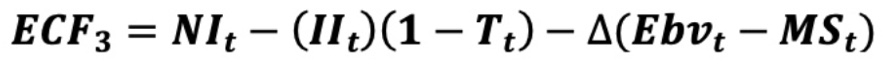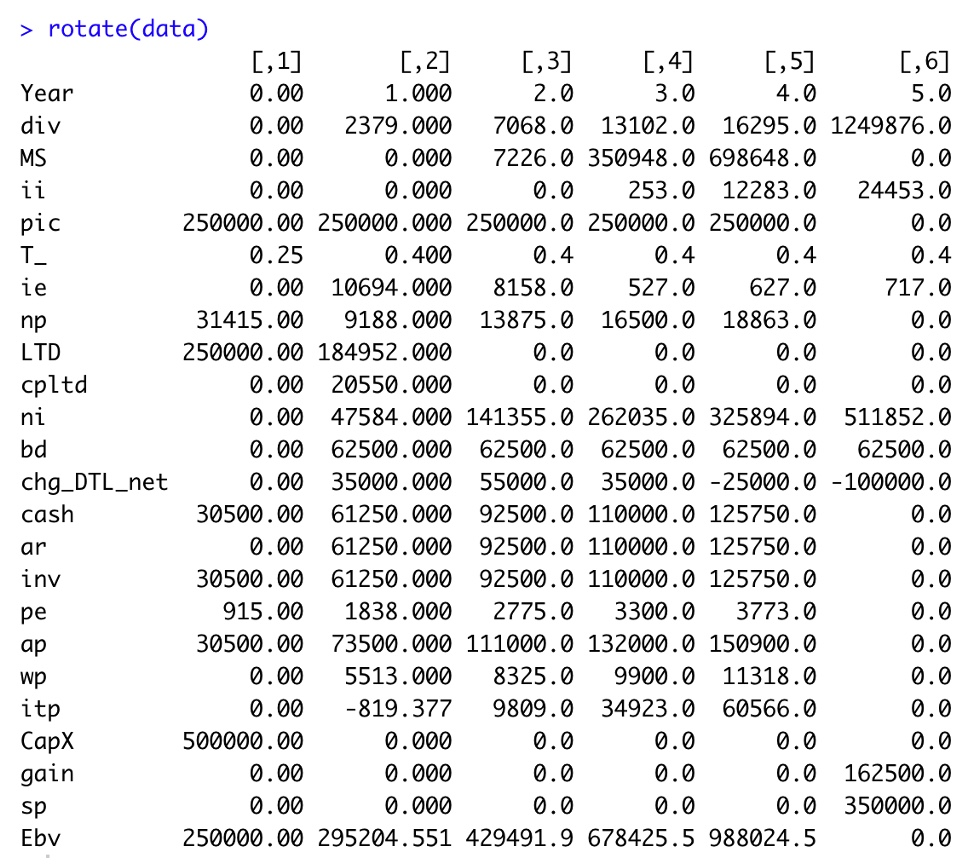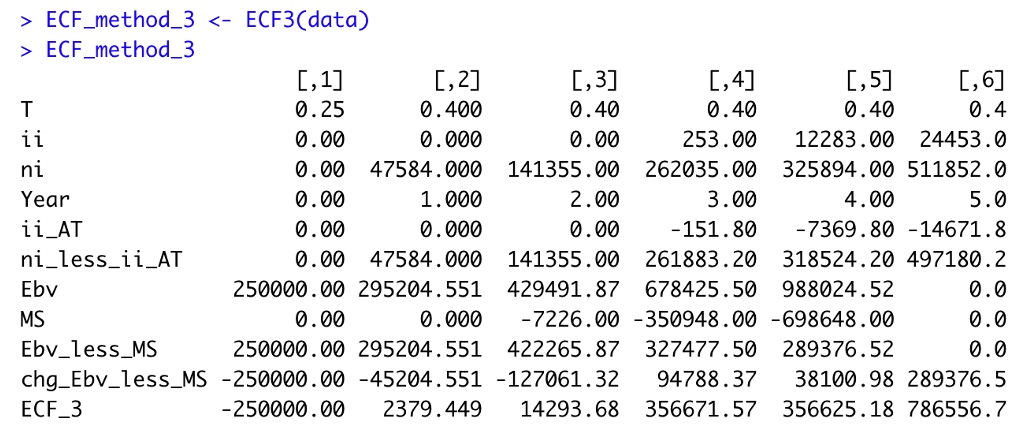Want to share your content on R-bloggers? click here if you have a blog, or here if you don't.

This represents Part 3 of a 4-part series relative to the calculation of Equity Cash Flow (ECF) using R.  If you missed Parts 1 and 2, be certain to reference them before proceeding. Content in this section builds off previously described information/data. Part 1 prior post is located here – Part 1 of 4 .
Part 2 prior post is located here – Part 2 of 4 . ‘ECF – Method 3’ is defined as follows:In words, Equity Cash Flow (ECF) equals Net Income (NI) less after-tax Interest Income less the change in the quantity ‘Equity Book Value (Ebv) minus Marketable Securities (MS).’ All terms in the equation are defined in the prior posts except for Ebv.

Reference details of the 5-year capital project’s fully integrated financial statements developed in R at the following link.  The R output is formatted in Excel and produced in a PDF file for ease of viewing.  Zoom the PDF for detail.

Financial Statements The Equity Book Value (Ebv) vector is added to the data tibble below.
```data <- data %>%
mutate(Ebv = c(250000, 295204.551, 429491.869, 678425.4966, 988024.52, 0 ))```
Though Ebv values are entered as known values, they are calculated in the text noted at the conclusion of this post.

View tibble.R function ECF3 defines the Equity Cash Flow (ECF) equation and its components.   ‘ECF – Method 3’ R function
```ECF3 <- function(a, b) {

library(tibble)

ECF3 <- a\$ni -a\$ii*(1-a\$T_) - ( a\$Ebv -lag(a\$Ebv, default=0) ) + ( a\$MS  - lag(a\$MS, default=0) )

ECF_3 <-     tibble(T              = a\$T_,
ii             = a\$ii,
ni             = a\$ni,
Year           = c(0:(length(ii)-1)),
ii_AT          = -ii*(1-T),
ni_less_ii_AT   = ni + ii_AT,
Ebv            = a\$Ebv,
MS             = -a\$MS,
Ebv_less_MS    = Ebv + MS,
chg_Ebv_less_MS  = - (Ebv_less_MS - lag(Ebv_less_MS, default=0) ) ,
ECF_3          = ni_less_ii_AT + chg_Ebv_less_MS )

ECF_3 <- rotate(ECF_3)

return(ECF_3)

}```
Run the R function and view the output.R Output formatted in Excel

ECF – Method 3ECF Method 3‘ agrees with the prior published methods each year.  Any differences are due to rounding error.

This ECF calculation example is taken from my newly published textbook, ‘Advanced Discounted Cash Flow (DCF) Valuation Using R.’ The above method is discussed in far greater detail along with development of the integrated financials using R as well 40+ advanced DCF valuation models – all of which are value-additivity compliant. Typical corporate finance texts do not teach this very important concept.

The text importantly clearly explains ‘why’ these ECF calculation methods are mathematically equivalent, though the equations may appear vastly different.

Reference my website for further details.

https://www.leewacc.com/

Next up, ‘ECF – Method 4‘ …

Brian K. Lee, MBA, PRM, CMA, CFA
Four (4) Different Ways to Calculate DCF Based ‘Equity Cash Flow (ECF)’ –  Part 3 of 4 was first posted on August 17, 2021 at 4:03 pm.